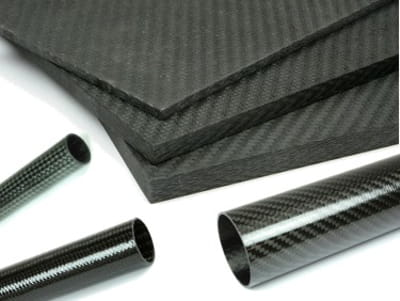# Carbon Fiber 101: Standard Modulus, Intermediate Modulus, High Modulus, and Ultra High ModulusMost carbon fiber is made from an industrial fiber called polyacrylonitrile fiber. The raw fiber is drawn and heated to high temperatures in an anaerobic environment to achieve its remarkable properties. In this quick article, we are going to cover the differences between standard modulus, intermediate modulus, high modulus, and ultra high modulus.

## What does Modulus (or Modulus of Elasticity) Mean

Modulus of Elasticity (E) is a measurement of the stiffness of a material, and is defined as the ratio of stress to strain when the material is deformed. On a stress strain curve, Elastic Modulus can be seen as the slope between two stress/strain points in the elastic region, and can be calculated as the difference in stress divided by the difference in strain.

Modulus of Elasticity, also known as Young's Modulus, is commonly used to predict or measure the stiffness of a tube or plate. Modulus is typically expressed in msi (million pounds per square inch) or Gigapascals. The higher the modulus, the stiffer the material (i.e. the greater the stress necessary to cause deformation).

## Standard Modulus Carbon Fiber

Standard Modulus carbon fibers are recognized as the most common and cost-effective fibers. These fibers exhibit a fiber modulus of 33-36 msi and can have tensile strength of 500-700 ksi

## Intermediate Modulus Carbon Fiber

Intermediate Modulus carbon fibers have a slightly higher Modulus of 43-47 msi and tensile strength 800 -1000 ksi

## High Modulus Carbon Fiber

High Modulus carbon fibers are created through specialized heat treatment processes which deliver much higher msi values around 57 msi, but at a reduced tensile strength of 640 ksi. This fiber will produce a part that is much stiffer, but not quite as strong.

## Ultra High Modulus

Ultra High Modulus carbon fibers are extremely stiff with values of 110msi, however tensile strength is even further degraded to 425 ksi.

By choosing between these options the engineer is best able to meet his stiffness, strength, and cost objectives when designing a carbon fiber composite part.